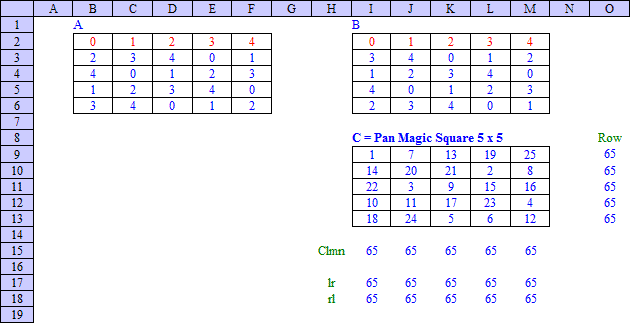Office Applications and Entertainment, Magic SquaresIndex About the Author

 3.6   Quinary Composition 3.6.1 Introduction Any number m = 0 ... 24 can be written as m = b1 + 5*b2 with bi = 0, 1, 2, 3, 4 for i = 1, 2 (Quinary representation). Consequently any Magic Square M of order 5 with the numbers 1 ... 25 can be written as M = B1 + 5 * B2 +  where the matrices B1 and B2 - further referred to as Quinary Squares - contain only the integers 0, 1, 2, 3 and 4. 3.6.2 Sudoku Comparable Squares Rows, columns and (pan) diagonals of the Quinary Squares B1 and B2 containing each the five integers 0, 1, 2, 3 and 4 will sum to the Magic Sum 10. Subject squares will be further referred to as Sudoku Comparable Squares. Sudoku Comparable Squares as described above can be obtained by applying the same equations as deducted in Section 3.2, however for a Magic Sum 10 and with the less strict restriction that only the elements of each row, column and diagonal should be different. An optimized guessing routine (SudSqr5a) produced 960 Sudoku Comparable Squares within 24,5 seconds, which are shown in Attachment 3.7.1. With an optional sub routine 'Check Pan Diagonals' activated 240 Sudoku Comparable Pan Magic Squares can be filtered from this collection, which are shown in Attachment 3.7.2. With an optional sub routine 'Check Associated' activated 64 Sudoku Comparable Associated Magic Squares can be filtered from this collection, which are shown in Attachment 3.7.3. With both options activated 16 Sudoku Comparable Associated Pan Magic Squares (Ultra Magic) can be filtered from this collection, which are shown in Attachment 3.7.4. A less strict collection of Sudoku Comparable Squares, with only the rows and columns containing the five distinct integers 0, 1, 2, 3 and 4 but also with the diagonals summing to the magic sum 10, could be produced with a comparable guessing routine (SudSqr5b) and are shown in Attachment 3.7.3. 3.6.3 Application and Results Vast amounts of (Pan) Magic Squares can be generated by selecting combinations of Quinary Squares (B1, B2) while ensuring that the resulting square M contains all integers 1 thru 25 (ref. CnstrSqrs5a): Based on the collection of 240 Quinary Pan Magic Squares (ref. Attachment 3.7.2) all 28800 Pan Magic Squares of the 5th order (ref. Attachment 5.2.6) were generated within 8 minutes; Based on the collection of 16 Quinary Ultra Magic Squares (ref. Attachment 3.7.4) all 128 Ultra Magic Squares of the 5th order (ref. Attachment 5.4.1) were generated within 4,7 seconds; Based on the collection of 64 Quinary Associated Magic Squares (ref. Attachment 3.7.3) 256 Associated Magic Squares of the 5th order could be generated within 13,9 seconds; Based on the collection of 960 Quinary Magic Squares (ref. Attachment 3.7.1) 57600 Magic Squares of the 5th order could be generated within 23 minutes; Based on the collection of 4288 Quinary Magic Squares (ref. Attachment 3.7.3) 143424 Magic Squares of the 5th order could be generated within 7,5 hours. The number of Simple Magic Squares found above are only a minor fraction of all Simple Magic Squares, as summarized in Section 5.8. A collection of 144 Pan Magic Squares (ref. Attachment 5.3.4) can be obtained by combining Sudoku Comparable Squares with following properties: B1: b1(1) = 0 and the contents of row 2, 3, 4 and 5 identical to row 1,     however every time two places to the left (24 ea); B2: b2(1) = 0, b2(2) = 1 and the contents of row 2, 3, 4 and 5 identical to row 1,     however every time two places to the right (6 ea). As none of these 144 squares can be obtained by means of rotation, reflection, row or column shift performed on one of the others, these squares can be used as a possible base for all 28800 Pan Magic Squares (ref. Attachment 5.2.6). 3.6.4 Sudoku Comparable Construction Method Based on above described principle, Pan Magic Squares of order 5 can be constructed by means of following procedure: Fill the first row of square A with the numbers 0, 1, 2, 3 and 4. While starting with 0 there are 4! = 24 possible combinations; Copy the first row of square A into the following rows of square A, however every time two places to the left. The numbers 0, 1, 2, 3 and 4 will occur now only one time in every row, column or (pan) diagonal; Fill the first row of square B with the numbers 0, 1, 2, 3 and 4. While starting with 0, 1 there are 3! = 6 possible combinations; Copy the first row of square B into the following rows of square B, however every time two places to the right. Construct the final square C by means of the matrix operation C = 5 * A + B + 1. Which can be realized by means of an Excel spreadsheet as shown below:The possible combinations of square A and B described above will result in 24 * 6 = 144 solutions, generated with routine MgcSqr5f in 10.25 seconds and shown in Attachment 5.3.4. Each of these 144 Pan Magic Squares will result in a unique Class Cn and finally in the 200 * 144 = 28800 possible Pan Magic Squares of the 5th order as shown in Attachment 5.2.6. Consequently this method is also very useful for higher order odd Pan Magic Squares.